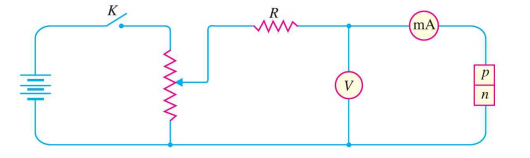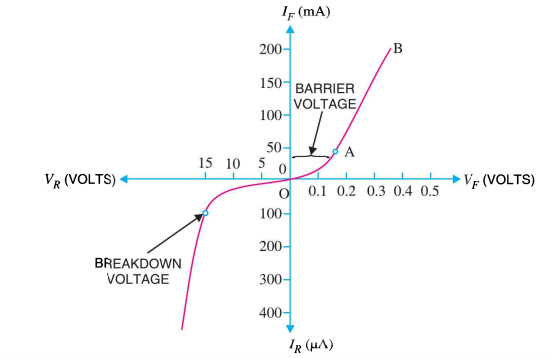# V-I Characteristics of PN Junction Diode

## V-I Characteristics of PN Junction Diode

Volt-ampere (V-I) characteristics of a pn junction or semiconductor diode is the curve between voltage across the junction and the current through the circuit.

Normally the voltage is taken along the x-axis and current along y-axis.

The circuit connection for determining the V-I characteristics of a pn junction is shown in the figure below.Fig.1: Circuit Connection for V-I characteristics of a pn junction

The characteristics can be explained under three cases , such as :

1. Zero bias
2. Forward bias
3. Reverse bias

### Case-1 : Zero Bias

In zero bias condition , no external voltage is applied to the pn junction i.e the circuit is open at K.

Hence, the potential barrier (ref :pn junction tutorial for better understanding) at the junction does not permit current flow.

Therefore, the circuit current is zero at V=0 V, as indicated by point O in figure below.Fig.2: V-I Characteristics of pn Junction

### Case-2 : Forward Bias

In forward biased condition , p-type of the pn junction is connected to the positive terminal and n-type is connected to the negative terminal of the external voltage.

This results in reduced potential barrier.

At some forward voltage  i.e 0.7 V for Si and 0.3 V for Ge, the potential barrier is almost eliminated and the current starts flowing in the circuit.

Form this instant, the current increases with the increase in forward voltage. Hence.  a curve OB is obtained with forward bias as shown in figure above.

From the forward characteristics, it can be noted that at first i.e. region OA , the current increases very slowly and the curve is non-linear. It is because in this region the external voltage applied to the pn junction is used in overcoming the potential barrier.

However, once the external voltage exceeds the potential barrier voltage, the  potential barrier is eliminated and the pn junction behaves as an ordinary conductor. Hence , the curve AB  rises very sharply with the increase in external voltage and the curve is almost linear.

### Case-3 : Reverse Bias

In reverse bias condition , the p-type of the pn junction is connected to the negative terminal and n-type is connected to the positive terminal of the external voltage.

This results in increased potential barrier at the junction.

Hence, the junction resistance becomes very high and as a result practically no current flows through the circuit.

However, a very small current of the order of μA , flows through the circuit in practice. This is knows as reverse saturation current(IS) and it is due to the minority carriers in the junction.

As we already know, there are few free electrons in p-type material and few holes in n-type material. These free electrons in p-type and holes in n-type are called minority carriers .

The reverse bias applied to the pn junction acts as forward bias to there minority carriers and hence, small current flows in the reverse direction.

If the applied reverse voltage is increased continuously, the kinetic energy of the minority carriers may become high enough to knock out electrons from the semiconductor atom.

At this stage breakdown of the junction may occur. This is characterized by a sudden increase of reverse current and a sudden fall of the resistance of barrier region. This may destroy the junction permanently.